Mathematical Models in Engineering

Published: 30 June 2020

# Hierarchical sliding mode attitude control of a spherical underwater exploring robot

Zhimin Liu1
1Shanghai Publishing and Printing College, Shanghai, 200093, China
Views 43

#### Abstract

Aiming at the attitude control of the spherical underwater robot, the kinematics and dynamics of the underactuated attitude control adjusting system are analyzed, and its dynamics model is established. By using the differential homeomorphism transformation, the model was divided into two subsystems. Based on the advantages of sliding mode variable structure control, this paper adopts the hierarchical sliding mode variable structure method to design the sliding mode of different variables respectively, and then designs the sliding mode of the whole system. The presented control scheme can solve the coupling problem well. The controller has small overshoot and can well suppress chattering phenomenon. The simulation results show that the designed control law can make the system converge quickly and control the attitude of the underwater spherical robot effectively.

#### Highlights

• The hierarchical sliding mode variable structure method was adopted to design the sliding mode of different variables.
• The presented control scheme can solve the coupling problem well.
• The controller has small overshoot and can well suppress chattering phenomenon.

## 1. Introduction

As a kind of special robots, spherical underwater robots have the following advantages: good compression performance, uncoupled hydrodynamic calculation, equal hydrodynamic parameters in each direction. Accordingly, spherical underwater vehicles have a broad prospect of application in underwater archaeological explorations, sea-floor geomorphic observation, aquaculture, and so on [1-3].

Underactuated system has many advantages in practical application. Due to the reduction of the actuators, the weight, cost and volume of the whole control system will be reduced. In a word, the research on the control method of underactuated system has important theoretical and application value . For underactuated vehicles, there were fewer independent motion control actuators than degrees of freedom (DOF) . It has been proven that the underactuated mechanical systems have second-order nonholonomic constraints . The attitude adjusting problem for underactuated underwater vehicles is of great challenge. The famous Brockett’s necessary condition shows that asymptotic stabilization of these underactuated UUVs cannot be achieved with any smooth or continuous time-invariant feedback controllers [7-9]. The underactuated control laws can be used to handle actuator failure for fully-actuated underwater vehicles or over-actuated underwater vehicles . Nowadays, the attitude adjusting problem of underwater vehicles and other rigid underactuated mechanical systems has made some achievements. Throughout the existing research results, the design methods of attitude adjusting controllers include backstepping method  and Lyapunov’s direct method , sliding mode control , adaptive control , saturated-state feedback control and model predictive control control . Among these methods, the sliding mode control (SMC) is one of the most effective means to handle the problem of motion control for underactuated underwater vehicles with external disturbance and model uncertainties, because it is natural strong robustness with regard to internal and external disturbance. Moreover, SMC has also the advantages of reduced order, decoupling, fast response, good dynamic characteristics and so on. Therefore, SMC can solve the attitude adjusting problem for underactuated underwater vehicles well.

The traditional SMC is an effective method for dealing with bounded uncertainty/disturbances and parasitic dynamics of nonlinear system. In this paper, a hierarchical sliding mode control method is proposed. The sliding planes of each layer of the method are asymptotically stable, which can drive the underactuated underwater robot to the desired state. Because the sliding mode control has the function of anti-interference, the designed controller in this paper has strong adaptability to all kinds of external disturbances.

## 2. Physical prototype of BYSQ-2

BYSQ-2 underwater spherical robot mainly consists of spherical catheter 1, Sleeve 2, long axis motor 3, weight pendulum 4 and short axis motor 5. Two motors placed perpendicular to each other, which can drive the weight pendulum to rotate to adjust the attitude. Physical prototype of robot is shown in Fig. 1.

Fig. 1Physical prototype of the robot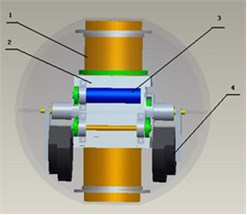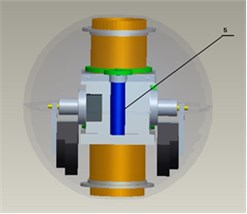If the components of the underwater spherical robot BYSQ-2 are all regarded as rigid bodies, then BYSQ-2 is a multirigid body composed of three rigid bodies. The spherical shell, catheter and propeller are fixedly connected with each other, which is rigid bodies ${B}_{1}$ with a total mass ${m}_{1}$; the sleeve is rigid body ${B}_{2}$ with a mass ${m}_{2}$; the two weightpendulums are called rigid body ${B}_{3}$ with a mass ${m}_{3}$.

## 3. Kinematic analysis of attitude control system

In order to analyze the motion of multi rigid body system of underwater spherical robot, the following four coordinate systems are established {0}, {1}, {2}, {3}, as shown in Fig. 2.

Fig. 2Coordinate system diagram of multi rigid body system of underwater spherical robot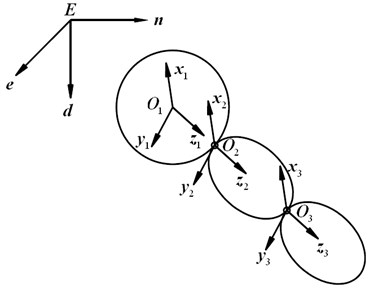The coordinate system {0} is an inertial reference system fixed to the earth, with the $En$ axis pointing north and the $Ed$ axis pointing vertically downward; the coordinate system {1} is a dynamic reference system fixed to the rigid body ${B}_{1}$, with the origin at the center of the ball, the ${O}_{1}{x}_{1}$ axis along the long axis, initially parallel to the coordinate system {0}, and the ${O}_{1}{x}_{1}$ axis coincides with the axis; the coordinate system {2} is fixed to the rigid body ${B}_{2}$, with the origin at the center of the ball, the ${O}_{2}{x}_{2}$ axis along the long axis, the coordinate system {3} is fixed to the rigid body ${B}_{3}$ and the origin is located at the center of the ball. At the beginning, the coordinate system {3} is parallel to the coordinate system {2} and the ${O}_{3}{x}_{3}$ axis coincides with the axis ${O}_{2}{x}_{2}$.

Because the Euler angles of the body coordinate system {1} and the relative inertia coordinate system {0} are respectively $\varphi$, $\theta$ and $\psi$, let:

1
$\left\{\begin{array}{l}{z}_{1}=\varphi ,\\ {z}_{2}=\theta ,\\ {z}_{3}=\psi .\end{array}\right\$

Then the angular velocity ${\omega }_{0}$ and acceleration ${\epsilon }_{0}$ of the coordinate system {1} relative to the coordinate system {0} are:

2
.

Because the angular velocity of the rigid body around the axis ${x}_{1}$ is $p$, the angular velocity in the axis ${O}_{1}{y}_{1}$ direction is $q$, and the angular velocity in the axis ${z}_{1}$ direction is $r$.

Let:

3
$\left\{\begin{array}{l}{z}_{4}=p,\\ {z}_{5}=q,\\ {z}_{6}=r.\end{array}\right\$

It can be concluded that the angular velocity ${\omega }_{1}$ and acceleration of the rigid body ${\epsilon }_{1}$ in the body coordinate system {1} are:

4

The relationship between the angular velocity of the rigid body ${B}_{1}$ in relative inertial coordinate system {0} and the body coordinate system {1} is as follows:

5
$\begin{array}{l}\stackrel{˙}{\varphi }=p+q\mathrm{s}\mathrm{i}\mathrm{n}\varphi \mathrm{t}\mathrm{a}\mathrm{n}\theta +r\mathrm{c}\mathrm{o}\mathrm{s}\varphi \mathrm{t}\mathrm{a}\mathrm{n}\theta ,\\ \stackrel{˙}{\theta }=q\mathrm{c}\mathrm{o}\mathrm{s}\varphi -r\mathrm{s}\mathrm{i}\mathrm{n}\varphi ,\\ \stackrel{˙}{\psi }=q\frac{\mathrm{s}\mathrm{i}\mathrm{n}\varphi }{\mathrm{c}\mathrm{o}\mathrm{s}\theta }+r\frac{\mathrm{c}\mathrm{o}\mathrm{s}\varphi }{\mathrm{c}\mathrm{o}\mathrm{s}\theta }.\end{array}$

Let the rotation angle of coordinate system {2} relative to axis ${O}_{1}{x}_{1}$ of coordinate system {1} be $\alpha$, The attitude transformation matrix can be obtained as:

6
$\left[\begin{array}{lll}1& 0& 0\\ 0& \mathrm{c}\mathrm{o}\mathrm{s}\alpha & -\mathrm{s}\mathrm{i}\mathrm{n}\alpha \\ 0& \mathrm{s}\mathrm{i}\mathrm{n}\alpha & \mathrm{c}\mathrm{o}\mathrm{s}\alpha \end{array}\right].$

Let the angular velocity of the rigid body ${B}_{2}$ around the axis ${O}_{2}{x}_{2}$ is ${z}_{7}$, then:

7
$\stackrel{˙}{\alpha }={z}_{4}+{z}_{7}.$

And let:

8
${z}_{8}=\alpha .$

Let the rotation angle of coordinate system {3} relative to axis ${O}_{2}{y}_{2}$ of coordinate system {2} be $\beta$, the angular velocity of the rigid body ${B}_{3}$ around the axis ${y}_{3}$ is ${z}_{9}$, then:

9
$\stackrel{˙}{\beta }={z}_{5}+{z}_{9}.$

The attitude transformation matrix can be obtained as:

10
$\left[\begin{array}{lll}1& 0& \mathrm{t}\mathrm{a}\mathrm{n}\beta \\ 0& 1& 0\\ 0& 0& \frac{1}{\mathrm{c}\mathrm{o}\mathrm{s}\beta }\end{array}\right].$

## 4. Dynamic analysis of attitude control system

According to the dynamic model equation of the underwater spherical robot established by Fossen , the dynamic equation of the rigid body ${B}_{1}$ when the propeller is not working can be obtained as follows:

11
$\begin{array}{l}{I}_{1x}\stackrel{˙}{p}+\left({I}_{1z}-{I}_{1y}\right)qr-\left(\stackrel{˙}{r}+pq\right){I}_{1xz}+\left({r}^{2}-{q}^{2}\right){I}_{1yz}+\left(pr-\stackrel{˙}{q}\right){I}_{1xy}={M}_{1},\\ {I}_{1y}\stackrel{˙}{q}+\left({I}_{1x}-{I}_{1z}\right)rp-\left(\stackrel{˙}{p}+qr\right){I}_{1xy}+\left({p}^{2}-{r}^{2}\right){I}_{1zx}+\left(pq-\stackrel{˙}{r}\right){I}_{1yz}={M}_{2y}^{\text{'}},\\ {I}_{1z}\stackrel{˙}{r}+\left({I}_{1y}-{I}_{1x}\right)pq-\left(\stackrel{˙}{q}+rp\right){I}_{1yz}+\left({q}^{2}-{p}^{2}\right){I}_{1xy}+\left(rq-\stackrel{˙}{p}\right){I}_{1xz}={M}_{2z}^{\text{'}},\end{array}$

where ${I}_{1x}$, ${I}_{1y}$, ${I}_{1z}$ are the moment of inertia of the rigid body ${B}_{1}$ around the ${O}_{1}{x}_{1}$ axis, ${O}_{1}{y}_{1}$ axis and ${O}_{1}{z}_{1}$ axis respectively. ${M}_{2y}^{\text{'}}$ and ${M}_{2z}^{\text{'}}$ are the components of the torque of the short axis motor acting on the rigid body on the ${O}_{1}{y}_{1}$ axis and ${O}_{1}{z}_{1}$ axis. ${I}_{1xy}$, ${I}_{1xz}$ and ${I}_{1yz}$ are the inertial products of rigid body ${B}_{1}$ to plane ${x}_{1}{o}_{1}{y}_{1}$, ${x}_{1}{o}_{1}{z}_{1}$ and ${y}_{1}{o}_{1}{z}_{1}$, respectively and:

12
$\begin{array}{l}{I}_{1x}={\int }_{\mathrm{\Omega }}\rho \left({y}^{2}+{z}^{2}\right)dxdydz,\\ {I}_{1y}={\int }_{\mathrm{\Omega }}\rho \left({x}^{2}+{z}^{2}\right)dxdydz,\\ {I}_{1z}={\int }_{\mathrm{\Omega }}\rho \left({x}^{2}+{y}^{2}\right)dxdydz,\end{array}$
13
$\begin{array}{l}{I}_{1xy}={\int }_{\mathrm{\Omega }}\rho xydxdydz,\\ {I}_{1xz}={\int }_{\mathrm{\Omega }}\rho xzdxdydz,\\ {I}_{1yz}={\int }_{\mathrm{\Omega }}\rho yzdxdydz.\end{array}$

Due to the symmetry of the structure, we have:

14
$\begin{array}{l}{I}_{1y}={I}_{1z},\\ {I}_{1xy}={I}_{1xz}={I}_{1yz}=0.\end{array}$

Since the values of ${I}_{x}$ are similar to those of ${I}_{y}$ and ${I}_{z}$, it is considered that:

15
${I}_{1x}={I}_{1y}={I}_{1z}.$

So Eq. (11) can be reduced to:

16
$\begin{array}{l}{I}_{1x}\stackrel{˙}{p}={M}_{1},\\ {I}_{1y}\stackrel{˙}{q}={M}_{2y}^{\text{'}},\\ {I}_{1z}\stackrel{˙}{r}={M}_{2z}^{\text{'}},\end{array}$

where ${I}_{2y}$ and ${I}_{2z}$ are the moment of inertia of the sleeve (rigid body ${B}_{2}$) around the ${O}_{2}{y}_{2}$ axis and ${O}_{2}{z}_{2}$ axis respectively.

Due to the regular shape of the sleeve, it can be approximately considered that:

17
${I}_{2y}={I}_{2z}={I}_{2yz},$

where ${I}_{2yz}$ is the moment of inertia of the sleeve (rigid body ${B}_{2}$) about any axis in the plane.

And we have:

18
$\begin{array}{l}{I}_{1x}\stackrel{˙}{p}={M}_{1},\\ \left({I}_{1y}+{I}_{2yz}\right)\stackrel{˙}{q}={M}_{2y}^{\text{'}},\\ \left({I}_{1z}+{I}_{2yz}\right)\stackrel{˙}{r}={M}_{2z}^{\text{'}}.\end{array}$

Let:

19
$\begin{array}{l}{I}_{y}^{\mathrm{\text{'}}}={I}_{1y}+{I}_{2y},\\ {I}_{z}^{\mathrm{\text{'}}}={I}_{1z}+{I}_{2z}.\end{array}$

Since:

20
${I}_{1y}={I}_{1z}.$

We have:

21
${I}_{y}^{\mathrm{\text{'}}}={I}_{z}^{\mathrm{\text{'}}}.$

Then the system model Eq. (16) can be rewritten as:

22
$\begin{array}{l}{I}_{1x}\stackrel{˙}{p}={M}_{1},\\ {I}_{y}^{\mathrm{\text{'}}}\stackrel{˙}{q}={M}_{2y}^{\text{'}},\\ {I}_{z}^{\mathrm{\text{'}}}\stackrel{˙}{r}={M}_{2z}^{\text{'}}.\end{array}$

In the coordinate system {3}, the torque of short axis motor can be expressed as:

23
$\left[\begin{array}{c}0\\ {M}_{2}\\ 0\end{array}\right].$

${M}_{2y}^{\text{'}}$ and ${M}_{2z}^{\text{'}}$ can be expressed as:

24

Then Eq. (22) can be reduced to:

25
$\begin{array}{l}\stackrel{˙}{p}=\frac{{M}_{1}}{{I}_{1x}},\\ \stackrel{˙}{q}=\frac{{M}_{2}\mathrm{c}\mathrm{o}\mathrm{s}\alpha }{{I}_{1y}+{I}_{2y}}\\ \stackrel{˙}{r}=\frac{{M}_{2}\mathrm{s}\mathrm{i}\mathrm{n}\alpha }{{I}_{1z}+{I}_{2z}}.\end{array},$

Let:

26

Then make the input transformation, let:

27
$\begin{array}{l}{\tau }_{1}={M}_{1},\\ {\tau }_{2}={M}_{2}.\end{array}$

Then the state equation of the spherical shell attitude control system is:

28
$\begin{array}{l}{\stackrel{˙}{z}}_{1}={z}_{4}+{z}_{5}\mathrm{s}\mathrm{i}\mathrm{n}{z}_{1}\mathrm{t}\mathrm{a}\mathrm{n}{z}_{2}+{z}_{6}\mathrm{c}\mathrm{o}\mathrm{s}{z}_{1}\mathrm{t}\mathrm{a}\mathrm{n}{z}_{2},\\ {\stackrel{˙}{z}}_{2}={z}_{5}\mathrm{c}\mathrm{o}\mathrm{s}{z}_{1}-{z}_{6}\mathrm{s}\mathrm{i}\mathrm{n}{z}_{1},\\ {\stackrel{˙}{z}}_{3}={z}_{5}\frac{\mathrm{s}\mathrm{i}\mathrm{n}{z}_{1}}{\mathrm{c}\mathrm{o}\mathrm{s}{z}_{2}}+{z}_{6}\frac{\mathrm{c}\mathrm{o}\mathrm{s}{z}_{1}}{\mathrm{c}\mathrm{o}\mathrm{s}{z}_{2}},\\ {\stackrel{˙}{z}}_{4}={k}_{1}{\tau }_{1},\\ {\stackrel{˙}{z}}_{5}={k}_{2}{\tau }_{2}\mathrm{c}\mathrm{o}\mathrm{s}{z}_{8},\\ {\stackrel{˙}{z}}_{6}={k}_{3}{\tau }_{2}\mathrm{s}\mathrm{i}\mathrm{n}{z}_{8},\\ {\stackrel{˙}{z}}_{7}=-2{k}_{4}{\tau }_{1},\\ {\stackrel{˙}{z}}_{8}={z}_{4}+{z}_{7}.\end{array}$

Further simplifying Eqs. (6-28), we can get:

29
$\begin{array}{l}{\stackrel{¨}{z}}_{1}-{\stackrel{¨}{z}}_{3}\mathrm{s}\mathrm{i}\mathrm{n}{z}_{2}-{\stackrel{˙}{z}}_{2}{\stackrel{˙}{z}}_{3}\mathrm{c}\mathrm{o}\mathrm{s}{z}_{2}={k}_{1}{\tau }_{1},\\ {\stackrel{¨}{z}}_{2}\mathrm{c}\mathrm{o}\mathrm{s}{z}_{1}+{\stackrel{¨}{z}}_{3}\mathrm{s}\mathrm{i}\mathrm{n}{z}_{1}\mathrm{c}\mathrm{o}\mathrm{s}{z}_{2}+{\stackrel{˙}{z}}_{1}{\stackrel{˙}{z}}_{3}\mathrm{c}\mathrm{o}\mathrm{s}{z}_{1}\mathrm{c}\mathrm{o}\mathrm{s}{z}_{2}-{\stackrel{˙}{z}}_{1}{\stackrel{˙}{z}}_{2}\mathrm{s}\mathrm{i}\mathrm{n}{z}_{1}-{\stackrel{˙}{z}}_{2}{\stackrel{˙}{z}}_{3}\mathrm{s}\mathrm{i}\mathrm{n}{z}_{1}\mathrm{s}\mathrm{i}\mathrm{n}{z}_{2}={k}_{2}{\tau }_{2}\mathrm{c}\mathrm{o}\mathrm{s}{z}_{8},\\ -{\stackrel{¨}{z}}_{2}\mathrm{s}\mathrm{i}\mathrm{n}{z}_{1}+{\stackrel{¨}{z}}_{3}\mathrm{c}\mathrm{o}\mathrm{s}{z}_{1}\mathrm{c}\mathrm{o}\mathrm{s}{z}_{2}-{\stackrel{˙}{z}}_{1}{\stackrel{˙}{z}}_{3}\mathrm{s}\mathrm{i}\mathrm{n}{z}_{1}\mathrm{c}\mathrm{o}\mathrm{s}{z}_{2}-{\stackrel{˙}{z}}_{1}{\stackrel{˙}{z}}_{2}\mathrm{c}\mathrm{o}\mathrm{s}{z}_{1}-{\stackrel{˙}{z}}_{2}{\stackrel{˙}{z}}_{3}\mathrm{c}\mathrm{o}\mathrm{s}{z}_{1}\mathrm{s}\mathrm{i}\mathrm{n}{z}_{2}={k}_{3}{\tau }_{2}\mathrm{s}\mathrm{i}\mathrm{n}{z}_{8},\\ {\stackrel{¨}{z}}_{8}=\left({k}_{1}-2{k}_{4}\right){\tau }_{1}.\end{array}$

Let:

30
$\left[\begin{array}{l}{\epsilon }_{11}\\ {\epsilon }_{21}\\ {\epsilon }_{31}\\ {\epsilon }_{41}\end{array}\right]=\left[\begin{array}{l}{z}_{1}\\ {z}_{2}\\ {z}_{3}\\ {z}_{8}\end{array}\right].$

Considering modeling error and external interference, from Eq. (29), we can obtain:

31

where ${d}_{1}$, ${d}_{2}$ and ${d}_{3}$ are modeling errors and external disturbances, and

${f}_{1}\left(\epsilon \right)={\epsilon }_{12}{\epsilon }_{22}\mathrm{t}\mathrm{a}\mathrm{n}{\epsilon }_{21}+{\epsilon }_{22}{\epsilon }_{32}\mathrm{s}\mathrm{i}\mathrm{n}{\epsilon }_{21}\mathrm{t}\mathrm{a}\mathrm{n}{\epsilon }_{21}+{\epsilon }_{22}{\epsilon }_{32}\mathrm{c}\mathrm{o}\mathrm{s}{\epsilon }_{21},$

${F}_{1}\left(\epsilon \right)={k}_{2}\mathrm{t}\mathrm{a}\mathrm{n}{\epsilon }_{21}\left(\mathrm{s}\mathrm{i}\mathrm{n}{\epsilon }_{11}\mathrm{c}\mathrm{o}\mathrm{s}{\epsilon }_{41}+\mathrm{c}\mathrm{o}\mathrm{s}{\epsilon }_{11}\mathrm{s}\mathrm{i}\mathrm{n}{\epsilon }_{41}\right),$
${F}_{2}\left(\epsilon \right)={k}_{2}\left(\mathrm{c}\mathrm{o}\mathrm{s}{\epsilon }_{11}\mathrm{c}\mathrm{o}\mathrm{s}{\epsilon }_{41}-\mathrm{s}\mathrm{i}\mathrm{n}{\epsilon }_{11}\mathrm{s}\mathrm{i}\mathrm{n}{\epsilon }_{41}\right),$
${F}_{3}\left(\epsilon \right)=\frac{{k}_{2}}{\mathrm{c}\mathrm{o}\mathrm{s}{\epsilon }_{21}}\left(\mathrm{s}\mathrm{i}\mathrm{n}{\epsilon }_{11}\mathrm{c}\mathrm{o}\mathrm{s}{\epsilon }_{41}+\mathrm{c}\mathrm{o}\mathrm{s}{\epsilon }_{11}\mathrm{s}\mathrm{i}\mathrm{n}{\epsilon }_{41}\right).$

Since the values of ${\epsilon }_{41}$ and ${\epsilon }_{42}$ are only two state variables needed in the design of control system, and there is no expected value, Eqs. (6-31) can be simplified to:

32
$\begin{array}{l}{\stackrel{˙}{\epsilon }}_{11}={\epsilon }_{12},\\ {\stackrel{˙}{\epsilon }}_{12}={f}_{1}\left(\epsilon \right)+{F}_{1}\left(\epsilon \right){\tau }_{2}+{k}_{1}{\tau }_{1}+{d}_{1},\\ {\stackrel{˙}{\epsilon }}_{21}={\epsilon }_{22},\\ {\stackrel{˙}{\epsilon }}_{22}={f}_{2}\left(\epsilon \right)+{F}_{2}\left(\epsilon \right){\tau }_{2}+{d}_{2},\\ {\stackrel{˙}{\epsilon }}_{31}={\epsilon }_{32},\\ {\stackrel{˙}{\epsilon }}_{32}={f}_{3}\left(\epsilon \right)+{F}_{3}\left(\epsilon \right){\tau }_{2}+{d}_{3}.\end{array}$

## 5. Control design

To design the control law conveniently, the system Eq. (32) is divided into two subsystems:

33

Then the back stepping sliding mode control law is designed for the two subsystems respectively.

Firstly, for the system the position error of the system is:

34
$\left\{\begin{array}{l}{e}_{2}={\epsilon }_{21}-{\epsilon }_{21d},\\ {e}_{3}={\epsilon }_{31}-{\epsilon }_{31d}.\end{array}\right\$

The sliding mode function can be designed as:

35
$\left\{\begin{array}{l}{s}_{2}={c}_{2}{e}_{2}+{\stackrel{˙}{e}}_{2},\\ {s}_{3}={c}_{3}{e}_{3}+{\stackrel{˙}{e}}_{3},\end{array}\right\$

where ${c}_{2}$ and ${c}_{3}$ are positive constants.

From Eq. (31), we have:

36
$\begin{array}{l}{\stackrel{˙}{e}}_{2}={\stackrel{˙}{\epsilon }}_{21}-{\stackrel{˙}{\epsilon }}_{21d}={\epsilon }_{22},\\ {\stackrel{¨}{e}}_{2}={\stackrel{¨}{\epsilon }}_{21}-{\stackrel{¨}{\epsilon }}_{21d}={f}_{2}\left(\epsilon \right)+{F}_{2}\left(\epsilon \right){\tau }_{2}+{d}_{2},\\ {\stackrel{˙}{e}}_{3}={\stackrel{˙}{\epsilon }}_{31}-{\stackrel{˙}{\epsilon }}_{31d}={\epsilon }_{32},\\ {\stackrel{¨}{e}}_{3}={\stackrel{¨}{\epsilon }}_{31}-{\stackrel{¨}{\epsilon }}_{31d}={f}_{3}\left(\epsilon \right)+{F}_{3}\left(\epsilon \right){\tau }_{2}+{d}_{3},\end{array}$

and

37
$\begin{array}{l}{s}_{2}={c}_{2}\left({\epsilon }_{21}-{\epsilon }_{21d}\right)+{\epsilon }_{22},\\ {\stackrel{˙}{s}}_{2}={c}_{2}\left({\stackrel{˙}{\epsilon }}_{21}-{\stackrel{˙}{\epsilon }}_{21d}\right)+{\stackrel{˙}{\epsilon }}_{22}={c}_{2}{\epsilon }_{22}+{f}_{2}\left(\epsilon \right)+{F}_{2}\left(\epsilon \right){\tau }_{2}+{d}_{2},\\ {s}_{3}={c}_{3}\left({\epsilon }_{31}-{\epsilon }_{31d}\right)+{\epsilon }_{32},\\ {\stackrel{˙}{s}}_{3}={c}_{3}\left({\stackrel{˙}{\epsilon }}_{31}-{\stackrel{˙}{\epsilon }}_{31d}\right)+{\stackrel{˙}{\epsilon }}_{22}={c}_{3}{\epsilon }_{32}+{f}_{3}\left(\epsilon \right)+{F}_{3}\left(\epsilon \right){\tau }_{2}+{d}_{3}.\end{array}$

If the following control laws are designed:

38
$\left\{\begin{array}{l}{\tau }_{21}=\frac{1}{{F}_{2}\left(\epsilon \right)}\left(-{c}_{2}{\epsilon }_{22}-{f}_{2}\left(\epsilon \right)-{D}_{2}\mathrm{s}\mathrm{g}\mathrm{n}\left({s}_{2}\right)\right),\\ {\tau }_{22}=\frac{1}{{F}_{3}\left(\epsilon \right)}\left(-{c}_{3}{\epsilon }_{32}-{f}_{3}\left(\epsilon \right)-{D}_{3}\mathrm{s}\mathrm{g}\mathrm{n}\left({s}_{3}\right)\right),\end{array}\right\$

where ${D}_{2}$ is the upper bound of ${d}_{2}$,${D}_{3}$ is the upper bound of ${d}_{3}$, sgn represented symbolic function, its definition is given by:

39

Substituting Eqs. (38) and (39) into Eq. (37),we have:

40
$\begin{array}{l}{\stackrel{˙}{s}}_{2}=-{D}_{2}\mathrm{s}\mathrm{g}\mathrm{n}\left({s}_{2}\right)+{d}_{2},\\ {\stackrel{˙}{s}}_{3}=-{D}_{3}\mathrm{s}\mathrm{g}\mathrm{n}\left({s}_{3}\right)+{d}_{3},\end{array}$

and

41
$\left\{\begin{array}{l}{s}_{2}{\stackrel{˙}{s}}_{2}={s}_{2}\left(-{D}_{2}\mathrm{s}\mathrm{g}\mathrm{n}\left({s}_{2}\right)+{d}_{2}\right)=-{D}_{2}\left|{s}_{2}\right|+{d}_{2}{s}_{2}\le 0,\\ {s}_{3}{\stackrel{˙}{s}}_{3}={s}_{3}\left(-{D}_{3}\mathrm{s}\mathrm{g}\mathrm{n}\left({s}_{3}\right)+{d}_{3}\right)=-{D}_{3}\left|{s}_{3}\right|+{d}_{3}{s}_{3}\le 0.\end{array}\right\$

Due to the coupling effect between the two subsystems in the system, it is not reasonable to add the two control laws easily, and we need to introduce the control law corresponding to the coupling effect.

The total desired control law is set to:

42
${\tau }_{2}={\tau }_{21}+{\tau }_{22}+{\tau }_{2h},$

where ${\tau }_{2h}$ is the coupled switching control law of the system in approach stage.

Now we construct the second sliding plane:

43
$S=C{s}_{2}+{s}_{3},$

where $C$ is a positive constant.

To get the coupled switching control law, the CLF is chosen as:

44
${V}_{1}=\frac{1}{2}{S}^{2},$

the first-order time-derivative of the CLF ${V}_{1}$ renders to:

45
${\stackrel{˙}{V}}_{1}=S\stackrel{˙}{S}=S\left(C{\stackrel{˙}{s}}_{2}+{\stackrel{˙}{s}}_{3}\right),$

substituting Eqs. (37) into Eq. (45), we have:

46
${\stackrel{˙}{V}}_{1}=S\left(\left(C{F}_{2}\left(\epsilon \right){\tau }_{22}+{F}_{3}\left(\epsilon \right){\tau }_{21}\right)+\left(C{F}_{2}\left(\epsilon \right)+{F}_{3}\left(\epsilon \right)\right){\tau }_{2h}\right).$

If the exponential approach law is taken for the system from the initial state to the switching surface, then:

47
$\left(C{F}_{2}\left(\epsilon \right){\tau }_{22}+{F}_{3}\left(\epsilon \right){\tau }_{21}\right)+\left(C{F}_{2}\left(\epsilon \right)+{F}_{3}\left(\epsilon \right)\right){\tau }_{2h}=-{p}_{1}\mathrm{s}\mathrm{g}\mathrm{n}\left(S\right)-{p}_{2}S,$

where ${p}_{1}$ and ${p}_{2}$ are positive constants.

From Eq. (47), we have:

48
${\tau }_{2h}=-\frac{1}{C{F}_{2}\left(\epsilon \right)+{F}_{3}\left(\epsilon \right)}\left(C{F}_{2}\left(\epsilon \right){\tau }_{22}+{F}_{3}\left(\epsilon \right){\tau }_{21}+{p}_{1}\mathrm{s}\mathrm{g}\mathrm{n}\left(S\right)+{p}_{2}S\right),$

and

49
50

Secondly, for system the position error of the system is:

51
${e}_{1}={\epsilon }_{11}-{\epsilon }_{11d}.$

The sliding mode function is chosen by the following equation:

52
${s}_{1}={c}_{1}{e}_{1}+{\stackrel{˙}{e}}_{1},$

where ${c}_{1}$ is a positive constant.

From Eq. (31), we have:

53
$\begin{array}{l}{\stackrel{˙}{e}}_{1}={\stackrel{˙}{\epsilon }}_{11}-{\stackrel{˙}{\epsilon }}_{11d}={\epsilon }_{12},\\ {\stackrel{¨}{e}}_{1}={\stackrel{¨}{\epsilon }}_{11}-{\stackrel{¨}{\epsilon }}_{11d}={f}_{1}\left(\epsilon \right)+{F}_{1}\left(\epsilon \right){\tau }_{2}+{k}_{1}{\tau }_{1},\end{array}$

and

54
$\left\{\begin{array}{l}{s}_{1}={c}_{1}\left({\epsilon }_{11}-{\epsilon }_{11d}\right)+{\epsilon }_{12},\\ {\stackrel{˙}{s}}_{1}={c}_{1}\left({\stackrel{˙}{\epsilon }}_{11}-{\stackrel{˙}{\epsilon }}_{11d}\right)+{\stackrel{˙}{\epsilon }}_{12}={c}_{1}{\epsilon }_{12}+{f}_{1}\left(\epsilon \right)+{F}_{1}\left(\epsilon \right){\tau }_{2}+{k}_{1}{\tau }_{1}.\end{array}\right\$

Let us definite the following Lyapunov function:

55
${V}_{2}={V}_{1}+\frac{1}{2}{s}_{1}^{2}.$

If the exponential approach law is taken for the system from the initial state to the switching surface, then:

56
${c}_{1}{\epsilon }_{12}+{f}_{1}\left(\epsilon \right)+{F}_{1}\left(\epsilon \right){\tau }_{2}+{k}_{1}{\tau }_{1}=-{p}_{1}^{\mathrm{\text{'}}}\mathrm{s}\mathrm{g}\mathrm{n}\left({s}_{1}\right)-{p}_{2}^{\mathrm{\text{'}}}{s}_{1},$

where ${p}_{1}^{\text{'}}$ and ${p}_{2}^{\text{'}}$ are positive constants.

From Eq. (56), the control input ${\tau }_{1}$ can be obtained as:

57
${\tau }_{1}=-\frac{1}{{k}_{1}}\left({p}_{1}^{\mathrm{\text{'}}}\mathrm{s}\mathrm{g}\mathrm{n}\left({s}_{1}\right)+{p}_{2}^{\mathrm{\text{'}}}{s}_{1}+{c}_{1}{\epsilon }_{12}+{f}_{1}\left(\epsilon \right)+{F}_{1}\left(\epsilon \right){\tau }_{2}\right),$

and

58

According to the aforementioned discussion, it is known that the control law Eqs. (50), (57) can drive arbitrary tracking errors to converge to the origin.

## 6. Simulation

According to the designed hierarchical sliding mode control law, we carry out some computer simulations by using the Simulink box to demonstrate the performance of the designed controller. The initial attitude value ($\varphi ,\theta ,\psi$) of the rigid body ${B}_{1}$ is ($\pi /2,\pi /4,\pi /4$). The desired attitude value (${\varphi }_{d},{\theta }_{d},{\psi }_{d}$) of the rigid body${B}_{1}$ is ($\pi /6,\pi /3,\pi /2$). The parameters are selected as follows: ${c}_{1}=\text{48}$, ${c}_{2}=\text{60}$, ${c}_{3}=\text{80}$, $C=\text{96}$, ${p}_{1}=\text{8}$, ${p}_{2}=\text{9}$, ${p}_{1}^{\mathrm{\text{'}}}=\text{7}$, ${p}_{2}^{\mathrm{\text{'}}}=\text{12}$.

The modeling errors and interferences are selected as follows: ${D}_{1}=$ 0.2, ${D}_{2}=$ 0.1, ${D}_{3}=$ 0.3.

Fig. 3Change of roll angle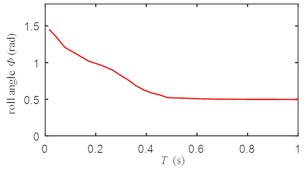Fig. 4Change of pitch angle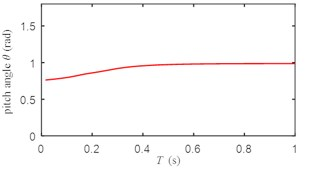Fig. 5Change of yaw angle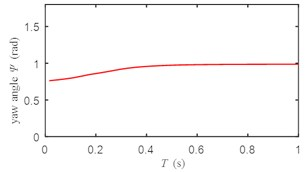Fig. 6Input torque change of long axis motor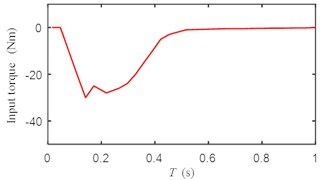Fig. 7Input torque change of short axis motor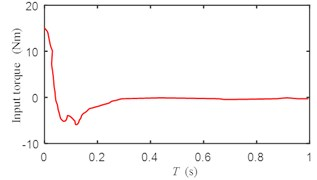According to Figs. 3-5, the response curves of roll angle, pitch angle and yaw angle have no oscillation, the rise time is less than 0.6 s, the transition time is less than 1 s, and the system has good dynamic performance.

According to Fig. 6, the input torque of the long axis motor has a small chattering, but does not affect the output of the system.

According to Fig. 7, the input torque of the short axis motor have no chatter.

In order to further test the performance of the controller, we carried out a circular experiment. The expected trajectory of the underwater spherical robot is an arc with a radius of 0.5 m, that is ${x}_{d}=$ 0.5 m, ${y}_{d}=$ 0.5 m, the initial value of ($x$, $y$, $\theta$) is (0, 0.5, 0). The value of $\theta$ is from 0 to $\pi /2$. The disturbance of the current can be expressed as ${\tau }_{w}=0.6+0.8\mathrm{s}\mathrm{i}\mathrm{n}\left(0.8t+\pi /4\right)$.

The simulation results are shown in Figs. 8-11.

Fig. 8Change of the yaw angle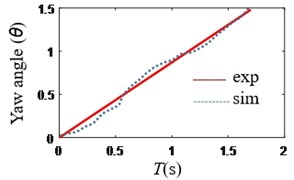Fig. 9Change of y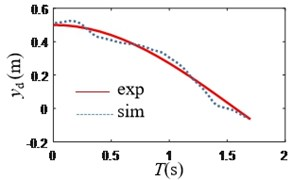Fig. 10Change of x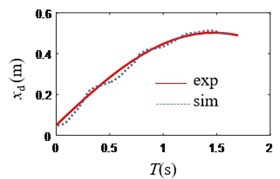Fig. 11Trajectory of the robot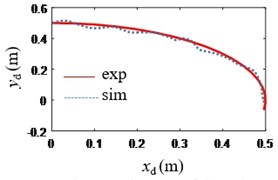According to Figs. 8-9, the absolute value of heading angle error is less than 0.2 rad, the absolute value of horizontal position error is less than 0.10 m, and the absolute value of vertical position error is less than 0.08 m.

According to Figs. 10-11, the trajectory of the underwater spherical robot is an arc with an approximate radius of 0.5 m.

Therefore, the experimental results show that the established dynamic control law based on this model are effective, which can realize the tracking of the motion track in the vertical plane, The controller has small overshoot and can well suppress chattering phnomenon and the control accuracy is ideal.

The above simulation results show the feasibility of the hierarchical sliding mode control law in the attitude adjusting control of the underwater spherical robot.

#### Acknowledgements

The authors would like to thank the support of China National Natural Science Foundation (51175048) for the research.